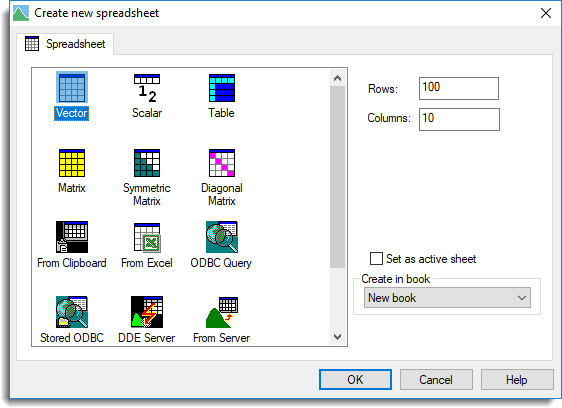1. Home
2. What Type of Spreadsheet can I Create?

# What Type of Spreadsheet can I Create?

In Genstat data are stored in different types of data structures, such as scalars, variates, factors and matrices. There are six different types of spreadsheets that can be used to display or manipulate data.This is the default type of spreadsheet and can contain one or more vector data structures (variates, texts and factors). Each column within the spreadsheet contains the values of one data structure and all the data structures must be the same length. By default, columns are variates, but they can easily be converted to another data type.

This type of spreadsheet can contain a single row of one or more scalars (single value).

This type of spreadsheet can contain tables. A table is a data structure that stores a multi-dimensional array of numbers where each dimension is classified by a factor. A spreadsheet can display tables with up to 9 dimensions. One-way tables that have the same classifying factor can be displayed on the same sheet, otherwise only a single table can be displayed on a sheet.

This type of spreadsheet can contain a single matrix.